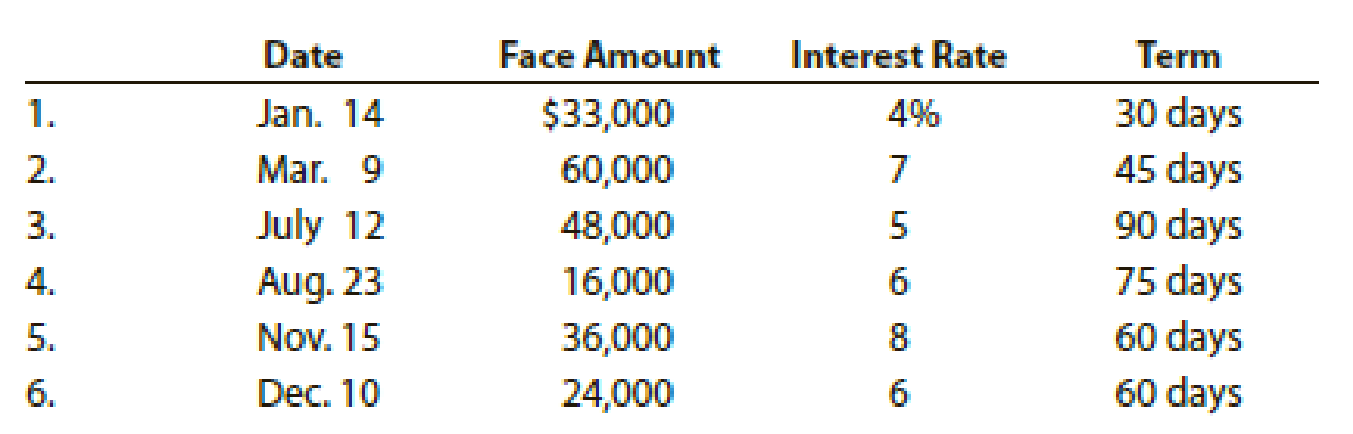# Gen-X Ads Co. produces advertising videos. During the current fiscal year, Gen-X Ads Co. received the following notes: Instructions 1. Determine for each note (a) the due date and (b) the amount of interest due at maturity, identifying each note by number. 2. Journalize the entry to record the dishonor of Note (3) on its due date. 3. Journalize the adjusting entry to record the accrued interest on Notes (5) and (6) on December 31. 4. Journalize the entries to record the receipt of the amounts due on Notes (5) and (6) in January and February.### Financial Accounting

14th Edition
Carl Warren + 2 others
Publisher: Cengage Learning
ISBN: 9781305088436

#### Solutions

Chapter
Section### Financial Accounting

14th Edition
Carl Warren + 2 others
Publisher: Cengage Learning
ISBN: 9781305088436
Chapter 9, Problem 4PB
Textbook Problem
57 views

## Gen-X Ads Co. produces advertising videos. During the current fiscal year, Gen-X Ads Co. received the following notes:Instructions 1. Determine for each note (a) the due date and (b) the amount of interest due at maturity, identifying each note by number. 2. Journalize the entry to record the dishonor of Note (3) on its due date. 3. Journalize the adjusting entry to record the accrued interest on Notes (5) and (6) on December 31. 4. Journalize the entries to record the receipt of the amounts due on Notes (5) and (6) in January and February.

(1)

To determine

Calculate (a) the due date and (b) the amount of interest due at maturity.

### Explanation of Solution

Note receivable: Note receivable refers to a written promise received by the creditor from the debtor in formal, for the amounts to be settled within a stipulated period of time. This written promise is issued by a debtor or borrower to the lender or creditor. Notes receivable is an asset of a business. Notes receivable often used for the credit periods of more than 60 days.

Due date: Due date is the maturity date on note, on due date the borrower is supposed to repay the face value of the note along with interest.

Interest on note: Interest on note is the amount charged on the principal value of note for the privilege of borrowing money. Interest is to be paid by the borrower and to be received by the lender.

Determine (a) the due date and (b) the amount of interest due at maturity.

 Due date Amount of interest due at maturity 1. February 13 $110 (1) 2. April 23$525 (2) 3. October10 $600 (3) 4. November 6$200 (4) 5. January 14 $480 (5) 6. February 8$240 (6)

Table (1)

Working note (1):

For note 1:

Calculate the amount of interest due at maturity.

Total interest=[Face amount ×Annual interest rate×Time in terms of year]=[$33,000×4%100×30 days360 days]=$110

Working note (2):

For note 2:

Calculate the amount of interest due at maturity.

Total interest=[Face amount ×Annual interest rate×Time in terms of year]=[$60,000×7%100×45 days360 days]=$525

Working note (3):

For note 3:

Calculate the amount of interest due at maturity.

Total interest=[Face amount ×Annual interest rate×Time in terms of year]=[$48,000×5%100×90 days360 days]=$600

Working note (4):

For note 4:

Calculate the amount of interest due at maturity

(2)

To determine

Journalize the dishonor of Note (3) on its due date.

(3)

To determine

Journalize the adjusting entry to record the accrued interest on Notes (5) and (6) on December 31.

(4)

To determine

Journalize the entries to record the receipt of the amounts due on Notes (5) and (6) in January.

### Still sussing out bartleby?

Check out a sample textbook solution.

See a sample solution

#### The Solution to Your Study Problems

Bartleby provides explanations to thousands of textbook problems written by our experts, many with advanced degrees!

Get Started

Find more solutions based on key concepts
Describe backup and recovery.

Accounting Information Systems

In what ways is economics a science?

Essentials of Economics (MindTap Course List)

What is an XBRL instance document?

Accounting Information Systems

PREFERRED STOCK RATE OF RETURN What will be the nominal rate of return on a perpetual preferred stock with a 10...

Fundamentals of Financial Management, Concise Edition (with Thomson ONE - Business School Edition, 1 term (6 months) Printed Access Card) (MindTap Course List)

What are the three major parts of a T account?

College Accounting, Chapters 1-27 (New in Accounting from Heintz and Parry)